﻿ 调和Bergman空间上大Hankel算子性质的研究 Study on Properties of Big Hankel Operator on Harmonic Bergman Space

Pure Mathematics
Vol.08 No.03(2018), Article ID:24811,5 pages
10.12677/PM.2018.83025

Study on Properties of Big Hankel Operator on Harmonic Bergman Space

Jing Yang

College of Mathematics and System Science, Shenyang Normal University, Shenyang LiaoningReceived: Apr. 18th, 2018; accepted: May 1st, 2018; published: May 10th, 2018ABSTRACT

This article mainly discusses some of the properties of the Big Hankel operator whose symbol is a radial function on the Bergman space. It constructs a series $\left\{{\phi }_{k}\right\}$ related to its symbolic function and obtains some conclusions about the nature of the big Hankel operator. The boundedness of the big Hankel operator is equivalent to the boundedness of $\left\{{\phi }_{k}\right\}$ . The compactness of the big Hankel operator converges to zero with $\left\{{\phi }_{k}\right\}$ , and the positivity of the big Hankel operator is equivalent to the bounded sequence with $\left\{{\phi }_{k}\right\}$ greater than zero.

Keywords:Big Hankel Operators, Harmonic Bergman Spaces, Boundedness, Compactness, Positivity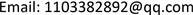1. 引言

$ℂ$ 为复平面， $D=\left\{z\in ℂ:|z|<1\right\}$$ℂ$ 中的单位开圆盘，使用dA定义D上的面积测度，所以规范D的面积是1，按照直角坐标和极坐标，有 $\text{d}A\left(z\right)=\frac{1}{\text{π}}\text{d}x\text{d}y=\frac{r}{\text{π}}\text{d}r\text{d}\theta$ ，其中 $z=x+iy$

$〈u,v〉={\int }_{D}u\overline{v}\text{d}A$

${L}^{2}\left(D,\text{d}A\right)$ 为一个Hilbert空间。

$\left(Qf\right)\left(z\right)=〈f,{R}_{z}〉={\int }_{D}f\left(w\right)\left({K}_{z}+\overline{{K}_{z}}-1\right)\text{d}A\left(w\right)=Pf+\overline{P\overline{f}}-Pf\left(0\right)$ .

$Uf\left(z\right)=\stackrel{˜}{f\left(z\right)}=f\left(\overline{z}\right)$$f\in {L}^{2}\left(D,\text{d}A\right)$ .

2. 主要结论

$\begin{array}{c}{\Gamma }_{\phi }f\left(z\right)=Q{M}_{\phi }\left(Uf\right)\\ ={\int }_{D}{M}_{\phi }\left(Uf\left(w\right)\right)R\left(z,w\right)\text{d}A\left(w\right)\\ ={\int }_{D}\phi \left(w\right)f\left(\overline{w}\right)\left({K}_{z}\left(w\right)+\overline{{K}_{z}\left(w\right)}-1\right)\text{d}A\left(w\right)\\ ={\int }_{D}\phi \left(w\right)f\left(\overline{w}\right){K}_{z}\left(w\right)\text{d}A\left(w\right)+{\int }_{D}\phi \left(w\right)f\left(\overline{w}\right)\overline{{K}_{z}\left(w\right)}\text{ }\text{d}A\left(w\right)\\ \text{\hspace{0.17em}}\text{\hspace{0.17em}}\text{ }\text{ }-{\int }_{D}\phi \left(w\right)f\left(\overline{w}\right)\text{d}A\left(w\right)\end{array}$

$\begin{array}{l}{\int }_{D}\phi \left(w\right)f\left(\overline{w}\right){K}_{z}\left(w\right)\text{d}A\left(w\right)\\ ={\int }_{D}\phi \left(w\right)f\left(\overline{w}\right)\left({\sum }_{k=0}^{\infty }\left(k+1\right){z}^{k}{\overline{w}}^{k}\right)\text{d}A\left(w\right)\\ ={\sum }_{k=0}^{\infty }{z}^{k}{\int }_{D}\phi \left(w\right){\overline{w}}^{k}\left(k+1\right)\left({\sum }_{k=0}^{\infty }{f}_{k}{\overline{w}}^{k}+{\sum }_{k=0}^{\infty }\stackrel{˜}{{f}_{k}}{w}^{k}\right)\text{d}A\left(w\right)\\ ={\sum }_{k=0}^{\infty }{z}^{k}{\int }_{D}\left[\phi \left(w\right)\left(k+1\right){\overline{w}}^{k}{\sum }_{k=0}^{\infty }{f}_{k}{\overline{w}}^{k}+\phi \left(w\right)\left(k+1\right){\overline{w}}^{k}{\sum }_{k=0}^{\infty }\stackrel{˜}{{f}_{k}}{w}^{k}\right]\text{d}A\left(w\right)\\ ={\phi }_{0}{f}_{0}+{\sum }_{k=0}^{\infty }{\phi }_{k}\stackrel{˜}{{f}_{k}}{z}^{k}\end{array}$

$\begin{array}{l}{\int }_{D}\phi \left(w\right)f\left(\overline{w}\right)\overline{{K}_{z}\left(w\right)}\text{ }\text{d}A\left(w\right)\\ ={\int }_{D}\phi \left(w\right)f\left(\overline{w}\right)\left({\sum }_{k=0}^{\infty }\left(k+1\right){\overline{z}}^{k}{w}^{k}\right)\text{d}A\left(w\right)\\ ={\sum }_{k=0}^{\infty }{z}^{k}{\int }_{D}\phi \left(w\right){w}^{k}\left(k+1\right)\left({\sum }_{k=0}^{\infty }{f}_{k}{\overline{w}}^{k}+{\sum }_{k=0}^{\infty }\stackrel{˜}{{f}_{k}}{w}^{k}\right)\text{d}A\left(w\right)\\ ={\sum }_{k=0}^{\infty }{z}^{k}{\int }_{D}\left[\phi \left(w\right)\left(k+1\right){w}^{k}{\sum }_{k=0}^{\infty }{f}_{k}{\overline{w}}^{k}+\phi \left(w\right)\left(k+1\right){\overline{w}}^{k}{\sum }_{k=0}^{\infty }\stackrel{˜}{{f}_{k}}{w}^{k}\right]\text{d}A\left(w\right)\\ ={\phi }_{0}\stackrel{˜}{{f}_{k}}+{\sum }_{k=0}^{\infty }{\phi }_{k}{f}_{k}{\overline{z}}^{k}\end{array}$

$\begin{array}{l}{\int }_{D}\phi \left(w\right)f\left(\overline{w}\right)\overline{{K}_{z}\left(w\right)}\text{ }\text{d}A\left(w\right)\\ ={\int }_{D}\phi \left(w\right)\left({\sum }_{k=0}^{\infty }{f}_{k}{\overline{w}}^{k}+{\sum }_{k=0}^{\infty }\stackrel{˜}{{f}_{k}}{w}^{k}\right)\text{d}A\left(w\right)={\phi }_{0}{f}_{0}+{\phi }_{0}\stackrel{˜}{{f}_{0}}\end{array}$

2.1. 大Hankel算子的有界性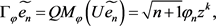，同理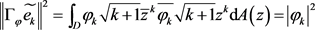，故有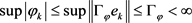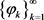有界。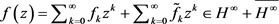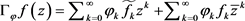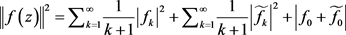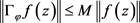2.2. 大Hankel算子的紧性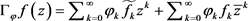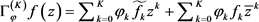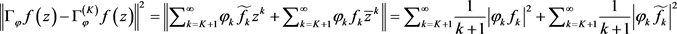，且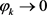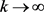时，故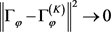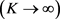因此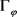为紧算子。

2.3. 大Hankel算子的正定性为正定。

Study on Properties of Big Hankel Operator on Harmonic Bergman Space[J]. 理论数学, 2018, 08(03): 203-207. https://doi.org/10.12677/PM.2018.83025

1. 1. Axler, S., Bourdon, P. and Ramey, W. (2001) Harmonic Function Theory. Springer, New York. https://doi.org/10.1007/978-1-4757-8137-3

2. 2. 王晓峰, 高崇志. 调和Bergman空间上特殊符号的Toeplitz算子[J]. 四川理工学院学报(自然科学版), 2006, 19(4): 1-4.

3. 3. Shu, Y.L. and Zhao, X.F. (2016) Positivity of Toeplitz Operators on Harmonic Bergman Space. Acta Mathematica Sinica, 32, 175-186. https://doi.org/10.1007/s10114-016-5138-7

4. 4. 黄辉斥. Bergman空间上小Hankel算子的代数性质(英文) [J]. 复旦学报(自然科学版), 2005, 44(3): 370-374+381.

5. 5. Osawa, T. (2006) Finite Rank Intermediate Hankel Operators and the Big Hankel Operator. International Journal of Mathematics and Mathematical Sciences, 2006, Article ID: 51705. https://doi.org/10.1155/IJMMS/2006/51705